Test: Power Electronics- 7

# Test: Power Electronics- 7 - Electrical Engineering (EE)

Test Description

## 10 Questions MCQ Test GATE Electrical Engineering (EE) 2024 Mock Test Series - Test: Power Electronics- 7

Test: Power Electronics- 7 for Electrical Engineering (EE) 2023 is part of GATE Electrical Engineering (EE) 2024 Mock Test Series preparation. The Test: Power Electronics- 7 questions and answers have been prepared according to the Electrical Engineering (EE) exam syllabus.The Test: Power Electronics- 7 MCQs are made for Electrical Engineering (EE) 2023 Exam. Find important definitions, questions, notes, meanings, examples, exercises, MCQs and online tests for Test: Power Electronics- 7 below.
Solutions of Test: Power Electronics- 7 questions in English are available as part of our GATE Electrical Engineering (EE) 2024 Mock Test Series for Electrical Engineering (EE) & Test: Power Electronics- 7 solutions in Hindi for GATE Electrical Engineering (EE) 2024 Mock Test Series course. Download more important topics, notes, lectures and mock test series for Electrical Engineering (EE) Exam by signing up for free. Attempt Test: Power Electronics- 7 | 10 questions in 30 minutes | Mock test for Electrical Engineering (EE) preparation | Free important questions MCQ to study GATE Electrical Engineering (EE) 2024 Mock Test Series for Electrical Engineering (EE) Exam | Download free PDF with solutions
 1 Crore+ students have signed up on EduRev. Have you?
Test: Power Electronics- 7 - Question 1

### A three phase bridge inverter is fed from a 500 V dc source. The inverter is operated in 180° conduction mode and it is supplying a purely resistive, star – connected load. The RMS value of the output (line) voltage is

Detailed Solution for Test: Power Electronics- 7 - Question 1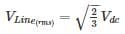= 0.816 × 500 = 408 V

Test: Power Electronics- 7 - Question 2

### In a three phase voltage source inverter operating in square wave mode, the output line voltage is free from

Detailed Solution for Test: Power Electronics- 7 - Question 2

The rms nth component of the line voltage is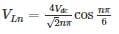where Vdc is the input source voltage for n = 3, line voltage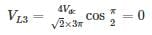Third harmonic component would not appear in the output

*Answer can only contain numeric values
Test: Power Electronics- 7 - Question 3

### In single pulse modulation of PWM inverter, 3rd harmonic can be eliminated if pulse width is equal to______ (in degrees)

Detailed Solution for Test: Power Electronics- 7 - Question 3

Pulse width for eliminating

nth harmonic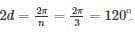*Answer can only contain numeric values
Test: Power Electronics- 7 - Question 4

A three – phase bridge inverter delivers power to a resistive load from a 450 V dc source. for a star connected load if 10Ω per phase. What is the load power for 180° conduction mode ______________ (in kW)

Detailed Solution for Test: Power Electronics- 7 - Question 4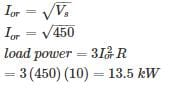Test: Power Electronics- 7 - Question 5

A 3 ϕ bridge inverter delivers power to a resistive load from a 400 V dc. For a star – connected load of 15 Ω per phase, determine load power and R.M.S value of thyristor current for 120° mode

Detailed Solution for Test: Power Electronics- 7 - Question 5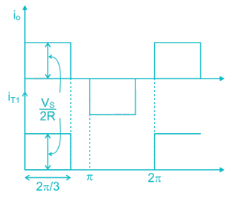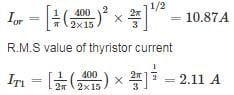load power = 3I2or R = 3 × (10.87)2 × 15 = 5.317kW

*Answer can only contain numeric values
Test: Power Electronics- 7 - Question 6

A 3 phase MOSFET inverter is connected between a balanced star connected resistive load with RL = 10 Ω and a 250 V dc supply. The inverter frequency is set at 33 Hz, and the switches have 120° conduction periods. Calculate the load power _______ (in kW)

Detailed Solution for Test: Power Electronics- 7 - Question 6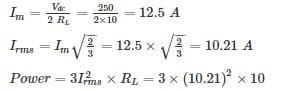= 3.13 kW

*Answer can only contain numeric values
Test: Power Electronics- 7 - Question 7

for a single pulse – width modulation of a single phase inverter, The distortion factor for pulse width of π/2 is ___________

Detailed Solution for Test: Power Electronics- 7 - Question 7

given, 2d = π/2

single pulse modulation output for pulse width 2d = π/2 is shown below.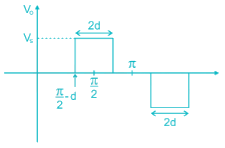from Fourier series, fundamental output voltage is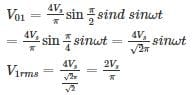from output voltage wave form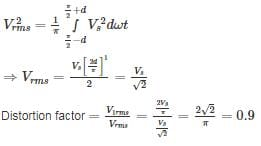Test: Power Electronics- 7 - Question 8

For a load of R = 10 Ω and L = 0.03 H, Find the RMS value of the fundamental component of inverter output current in case inverter of part Quasi-square output with 120° pulse width. The source voltage is 230 V and frequency 50 Hz

Detailed Solution for Test: Power Electronics- 7 - Question 8

Concept:

The output voltage is controlled by varying the pulse-width 2d. This shape of the output voltage is called Quasi-square wave.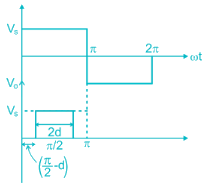Fourier series of this wave from is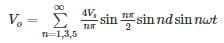Fundamental component of output voltage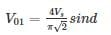Calculation:
Load impedance at Fundamental Frequency is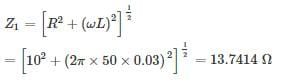For Quasi-square wave or single-pulse modulated wave where pulse width, 2d = 120° or d = 60°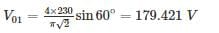∴ R.M.S value of fundamental current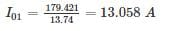*Answer can only contain numeric values
Test: Power Electronics- 7 - Question 9

The inverter is operated at 50 Hz. In 180° square wave mode. Find the peak to peak inductor i0 in the circuit shown in figure.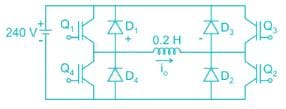Detailed Solution for Test: Power Electronics- 7 - Question 9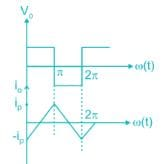at, t = 0, i0 = -ip and t = t1, i0 = ip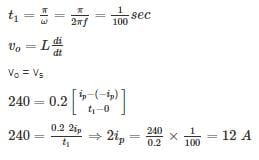*Answer can only contain numeric values
Test: Power Electronics- 7 - Question 10

For the symmetrical two pulse modulation with pulse width 36°. Find the percentage of 3rd harmonic in the output voltage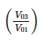is_______

Detailed Solution for Test: Power Electronics- 7 - Question 10

Concept:

In multiphase pulse modulation, several equidistant pulses per half cycle are used.

Fourier series of multiple pulse modulation is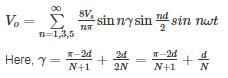N = pulse per half cycle

Calculation: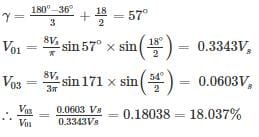## GATE Electrical Engineering (EE) 2024 Mock Test Series

23 docs|285 tests
Information about Test: Power Electronics- 7 Page
In this test you can find the Exam questions for Test: Power Electronics- 7 solved & explained in the simplest way possible. Besides giving Questions and answers for Test: Power Electronics- 7, EduRev gives you an ample number of Online tests for practice

## GATE Electrical Engineering (EE) 2024 Mock Test Series

23 docs|285 tests